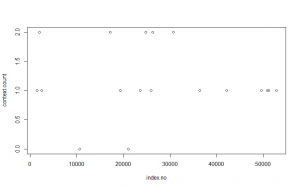# Mixed Results with the Aeneid

Code A

I must confess to getting a bit of a late start on this week’s blog post (busy week), and as a result I have found myself stuck on a particular line of the chunking code that I have yet to trial-and-error my way through. The 12 book (read: chapter) divisions of the Aeneid are listed as “Liber I, Liber II, Liber III, etc.”, and I can’t quite get the grep function (which I admittedly still do not fully understand) to mark these headings. I believe that the line of code as I have it (bolded below) indicates the phrase “LIBER + (some combination of Roman numerals”, but even so R comes back with 23 hits instead of the expected 12.

What I had intended to do was to track the occurrences of “virtus” (~manly martial virtuous excellence) and “pius” (~reverent toward the gods and one’s family and duty), both of which are major themes in the Aeneid. Perhaps I will be able to do so once I figure out what’s tripping me up with the grep function. Again, apologies for not coming to Dr. Ravina with this sooner.

Aeneid.lines.scan <- scan(
“~/Education/Emory/Coursework/Digital Humanities Methods/RStudios Practice/Aeneid Raw Text.txt”,
what=”character”, sep=”\n”) # Scan Aeneid Raw Text

start.line <-
which(Aeneid.lines.scan==”PUBLI VERGILI MARONIS”)
end.line <- which(Aeneid.lines.scan==”vitaque cum gemitu fugit indignata sub umbras.”)

poem.lines <- Aeneid.lines.scan[start.line : end.line]

end.lines <- c(end.lines, length(poem.lines))

Aeneid.df <- data.frame(“start” = start.lines, “end”=end.lines, “text”=NA)
i <- 1
for (i in 1:length(Aeneid.df\$end))
{Aeneid.df\$text[i] <- paste(poem.lines[Aeneid.df\$start[i]:Aeneid.df\$end[i]], collapse = ” “)} View(Aeneid.df)
Aeneid.df\$virtus <-
str_count(string = Aeneid.df\$text, pattern = “\\Wvirtus\\W|\\WVirtus\\W”)

Aeneid.df\$book <- seq(1,12,1)
plot(Aeneid.df\$book, Aeneid.df\$virtus)

Aeneid.df\$pius <-
str_count(string = Aeneid.df\$text, pattern = “\\Wpius\\W|\\WPius\\W”)

Aeneid.df\$book <- seq(1,12,1)
plot(Aeneid.df\$book, Aeneid.df\$pius)

Code B

I had more success dealing with the KWIC analysis (although I should point out that in both this and the previous set of coding, I am still hampered by my ignorance of stemming and NLTK for Latin. Here I looked at the context in which one found the word “pius” with either “Aeneas” (to whom the epithet is often given) or “At” (meaning “but”, and something that I noticed appeared in a number of lines with “pius”).

poem.total <- paste(poem.lines, collapse=” “)
length(poem.total)

nchar(poem.total)

poeml.total <- tolower(poem.total)

poem.words <- unlist(str_split(poem.total, “\\W”))
length(poem.words)

poem.words <- poem.words[which(poem.words!=””)]
length(poem.words)

locations.kwic <- which(poem.words==’pius’)
start.kwic <- locations.kwic – 5
end.kwic <- locations.kwic + 5
start.kwic <- ifelse(start.kwic>0, start.kwic, 0)
end.kwic <- ifelse(end.kwic<length(poem.words),
end.kwic, length(poem.words))

KWIC.df <- data.frame(“start” = start.kwic, “end” = end.kwic, “text” = NA)

i <- 1
for (i in 1:length(KWIC.df\$start)){
text <- poem.words[KWIC.df\$start[i]:KWIC.df\$end[i]]
KWIC.df\$text[i] <- paste(text, collapse = ” “)
}

view(text)

index.no <- which(poem.words==’pius’)
context.count <- str_count(KWIC.df\$text, “Aeneas|At”)
plot(index.no, context.count)